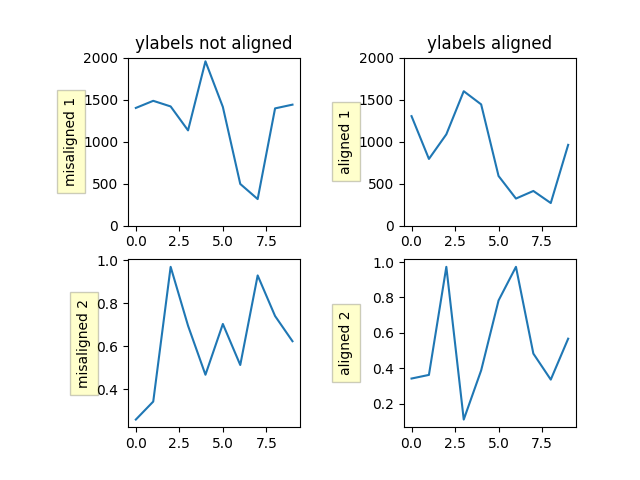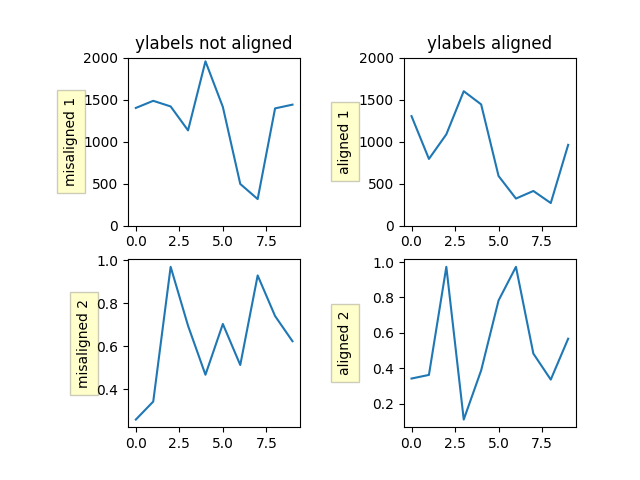# Align y-labels¶

Two methods are shown here, one using a short call to Figure.align_ylabels and the second a manual way to align the labels.

import numpy as np
import matplotlib.pyplot as plt

def make_plot(axs):
box = dict(facecolor='yellow', pad=5, alpha=0.2)

# Fixing random state for reproducibility
np.random.seed(19680801)
ax1 = axs[0, 0]
ax1.plot(2000*np.random.rand(10))
ax1.set_title('ylabels not aligned')
ax1.set_ylabel('misaligned 1', bbox=box)
ax1.set_ylim(0, 2000)

ax3 = axs[1, 0]
ax3.set_ylabel('misaligned 2', bbox=box)
ax3.plot(np.random.rand(10))

ax2 = axs[0, 1]
ax2.set_title('ylabels aligned')
ax2.plot(2000*np.random.rand(10))
ax2.set_ylabel('aligned 1', bbox=box)
ax2.set_ylim(0, 2000)

ax4 = axs[1, 1]
ax4.plot(np.random.rand(10))
ax4.set_ylabel('aligned 2', bbox=box)

# Plot 1:
fig, axs = plt.subplots(2, 2)
fig.subplots_adjust(left=0.2, wspace=0.6)
make_plot(axs)

# just align the last column of axes:
fig.align_ylabels(axs[:, 1])
plt.show()See also

Figure.align_ylabels and Figure.align_labels for a direct method of doing the same thing. Also Aligning Labels

Or we can manually align the axis labels between subplots manually using the set_label_coords method of the y-axis object. Note this requires we know a good offset value which is hardcoded.

fig, axs = plt.subplots(2, 2)
fig.subplots_adjust(left=0.2, wspace=0.6)

make_plot(axs)

labelx = -0.3  # axes coords

for j in range(2):
axs[j, 1].yaxis.set_label_coords(labelx, 0.5)

plt.show()